# Statistics - (Variance|Dispersion|Mean Square) (MS)

The variance shows how widespread the individuals are from the average.

The variance is how much that the estimate varies around its average.

It's a measure of consistency. A very large variance means that the data were all over the place, while a small variance (relatively close to the average) means that the majority of the data are closed.

See:

## Formula

$$\begin{array}{rrl} Variance & = & \frac\sum_{i=1}^{\href{sample_size}{N}}{(\href{raw_score}{X}_i- \href{mean}{\bar{X}})^2}}\href{degree_of_freedom}{\text{Degree of Freedom}}} \\ & = & \frac\sum_{i=1}^{\href{sample_size}{N}}{(\href{Deviation Score}{\text{Deviation Score}}_i)^2}}\href{degree_of_freedom}{\text{Degree of Freedom}}} \\ & = & (\href{Standard_Deviation}{\text{Standard Deviation}})^2 \end{array}$$

where:

$$Var(X + Y) = Var(X) + Var(Y) + 2 Cov(X, Y)$$ where:

## Computation

### Python

units = [7, 10, 9, 4, 5, 6, 5, 6, 8, 4, 1, 6, 6]

def units_average(units):
average = sum(units) / len(units)
return average

def units_variance(units,average):
diff = 0
for unit in units:
diff += (unit - average) ** 2
return diff / len(units)

print units_variance(units, units_average(units))

5



Recommended Pages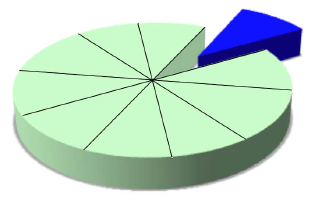(Statistics|Data Mining) - (K-Fold) Cross-validation (rotation estimation)

Cross-validation, sometimes called rotation estimation is a resampling validation technique for assessing how the results of a statistical analysis will generalize to an independent new data set. This...Data Mining - (Function|Model)

The model is the function, equation, algorithm that predicts an outcome value from one of several predictors. During the training process, the models are build. A model uses a logic and one of several...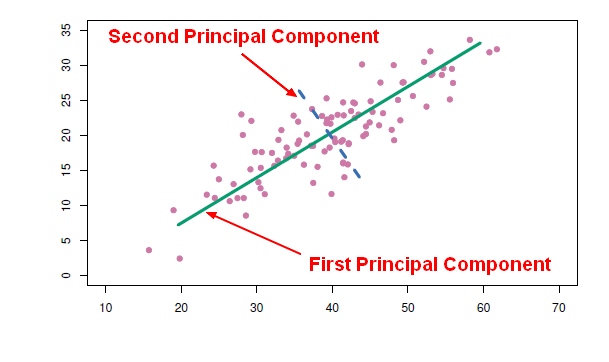Data Mining - Principal Component (Analysis|Regression) (PCA|PCR)

Principal Component Analysis (PCA) is a feature extraction method that use orthogonal linear projections to capture the underlying variance of the data. By far, the most famous dimension reduction approach...Data Mining - Random forest

Random forest (or random forests) is a trademark term for an ensemble classifier that consists of many decision trees and outputs the class that is the mode of the classes output by individual trees. ...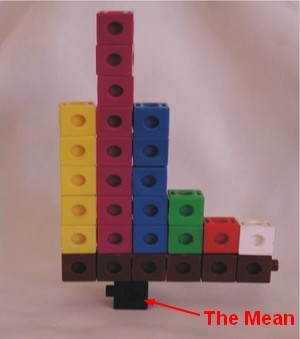Distribution - (Mean|Average) (M| | )

The average is a measure of center that statisticians call the mean. To calculate the mean, you add all numbers and divide the total by the number of numbers (N). The mean is not resistant. The...Machine Learning - (Overfitting|Overtraining|Robust|Generalization) (Underfitting)

A learning algorithm is said to overfit if it is: more accurate in fitting known data (ie training data) (hindsight) but less accurate in predicting new data (ie test data) (foresight) Ie the model...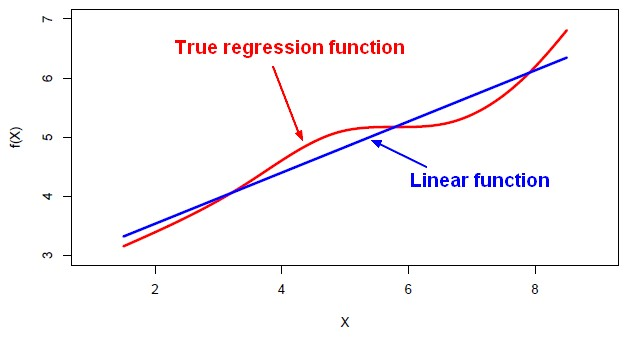Machine Learning - Linear (Regression|Model)

Linear regression is a regression method (ie mathematical technique for predicting numeric outcome) based on the resolution of linear equation. This is a classical statistical method dating back more...Machine learning - Bootstrap aggregating (bagging)

Bootstrap aggregating (bagging) is a machine learning ensemble meta-algorithm designed to improve the stability and accuracy of machine learning algorithms used in statistical classification and regression....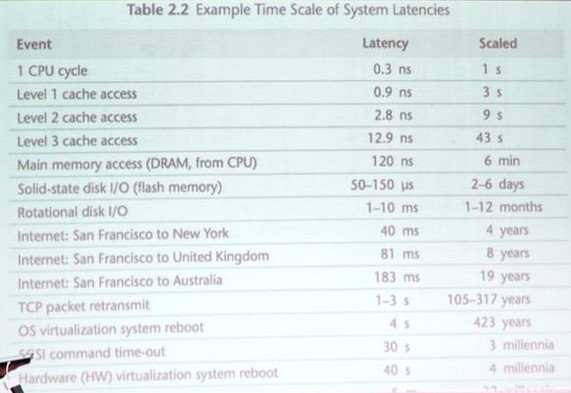Performance - (Latency|Response time|Running Time)

Latency is a performance metric also known as Response time. Latency (Response Time) is the amount of time take a system to process a request (ie to first response) from the outside or not, remote or...R - Variance

The variance calculus in R